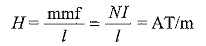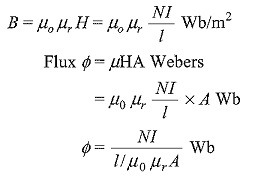## Series Magnetic Circuit:

A series magnetic circuit is analogous to a series electric circuit. Kirchhoff s laws are applicable to magnetic circuits also. Consider a ring specimen having a magnetic path of l meters, area of cross-section (A)m2 with a mean radius of R meters having a coil of N turns carrying I amperes wound uniformly as shown in Fig. 10.26. MMF is responsible for the establishment of flux in the magnetic medium. This mint acts along the magnetic lines of force. The flux produced by the circuit is given byThe magnetic field intensity of the ring is given byWhere l is the mean length of the magnetic path and is given by 2πR.

Flux densityNI is the mint of the magnetic circuit, which is analogous to emf in electric circuit. l0μrA is the reluctance of the Series Magnetic Circuit which is analogous to resistance in electric circuit.

Scroll to Top